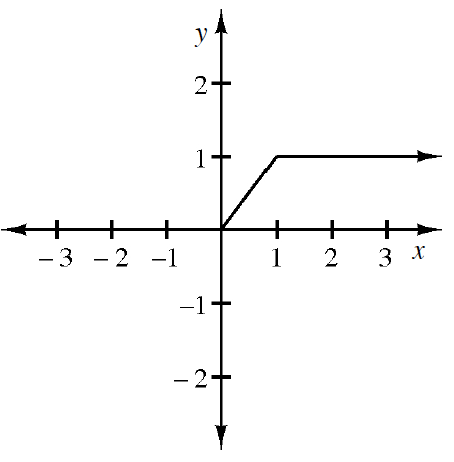### Home > APCALC > Chapter 1 > Lesson 1.4.1 > Problem1-138

1-138.

A portion of the graph of $y = f(x)$ is given at right:

1. Sketch the rest of the graph if $f$ is even and calculate the area under the curve for $−3 ≤ x ≤ 3$.

If a function is even, it is symmetric about the $y$-axis.

2. Sketch the rest of the graph if $f$ is odd and calculate the area under the curve for $−3 ≤ x ≤ 3$.

If a function is odd, it also has $180^\circ$ rotational symmetry about the origin.

Use the eTool below to visualize this problem.
Click the link at right for the full version of the eTool: Calc 1-138 HW eTool